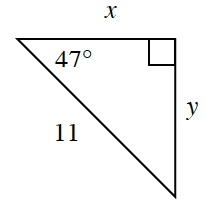### Home > INT2 > Chapter 4 > Lesson 4.2.1 > Problem4-62

4-62.

You now have multiple trig tools to determine missing side lengths of triangles. For the triangle below, determine the values of $x$ and $y$. Your Triangle Graphic Organizer might help. Which tools did you use?Use either sine or cosine to determine the length of one of the missing legs.

Then, use the Pythagorean Theorem or any of the trig ratios to determine the length of the other leg.